Home‎ > ‎

### Alphabet Codes

This is officially termed the ICAO (International Civil Aviation Organization) code. The ICAO is a United Nations department. Initially used by the US military, the code has since gained pervasive adoption by the airlines and is used throughout all civil aviation.
`    A=Alpha    B=Bravo    C=Charlie    D=Delta    E=Echo    F=Foxtrot    G=Golf    H=Hotel    I=India    J=Juliet    K=Kilo    L=Lima    M=Mike    N=November    O=Oscar    P=Papa    Q=Quebec    R=Romeo    S=Sierra    T=Tango    U=Uniform    V=Victor    W=Whiskey    X=X-ray    Y=Yankee    Z=Zulu`

### Maritime Signal Flags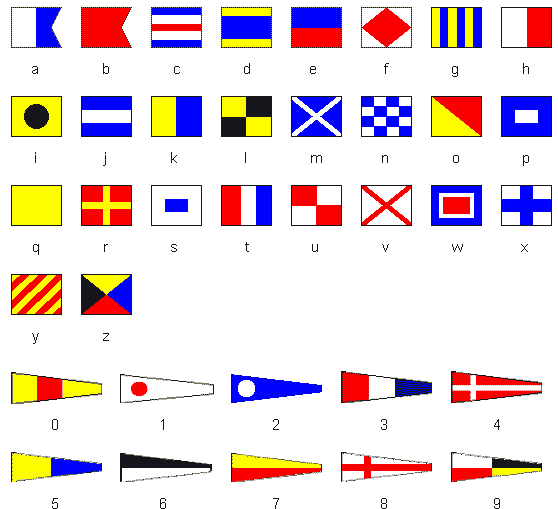### Semaphore Flag Signals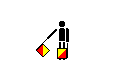A and 1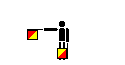B and 2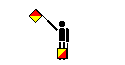C and 3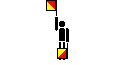D and 4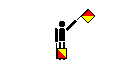E and 5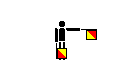F and 6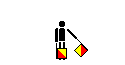G and 7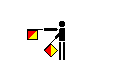H and 8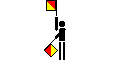I and 9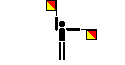J and Alphabetic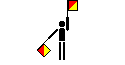K and 0 (zero)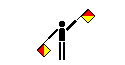L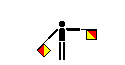M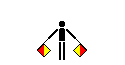N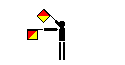O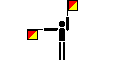P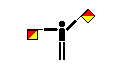Q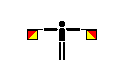R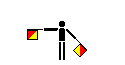S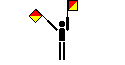T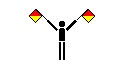U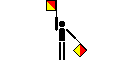V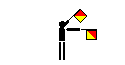W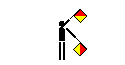X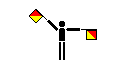Y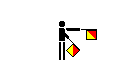Z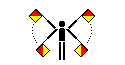Error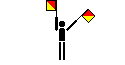Numerical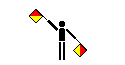Annul